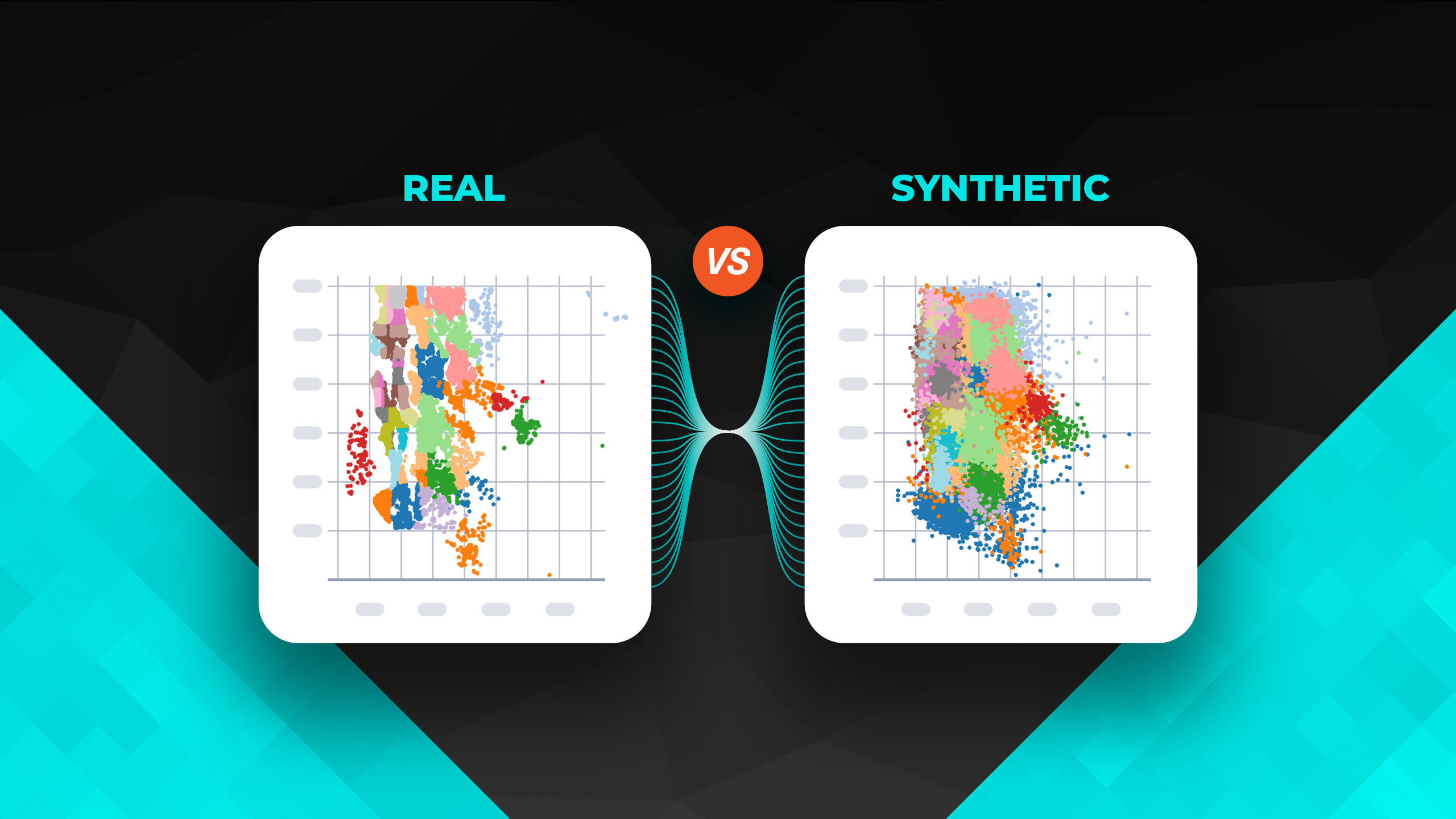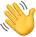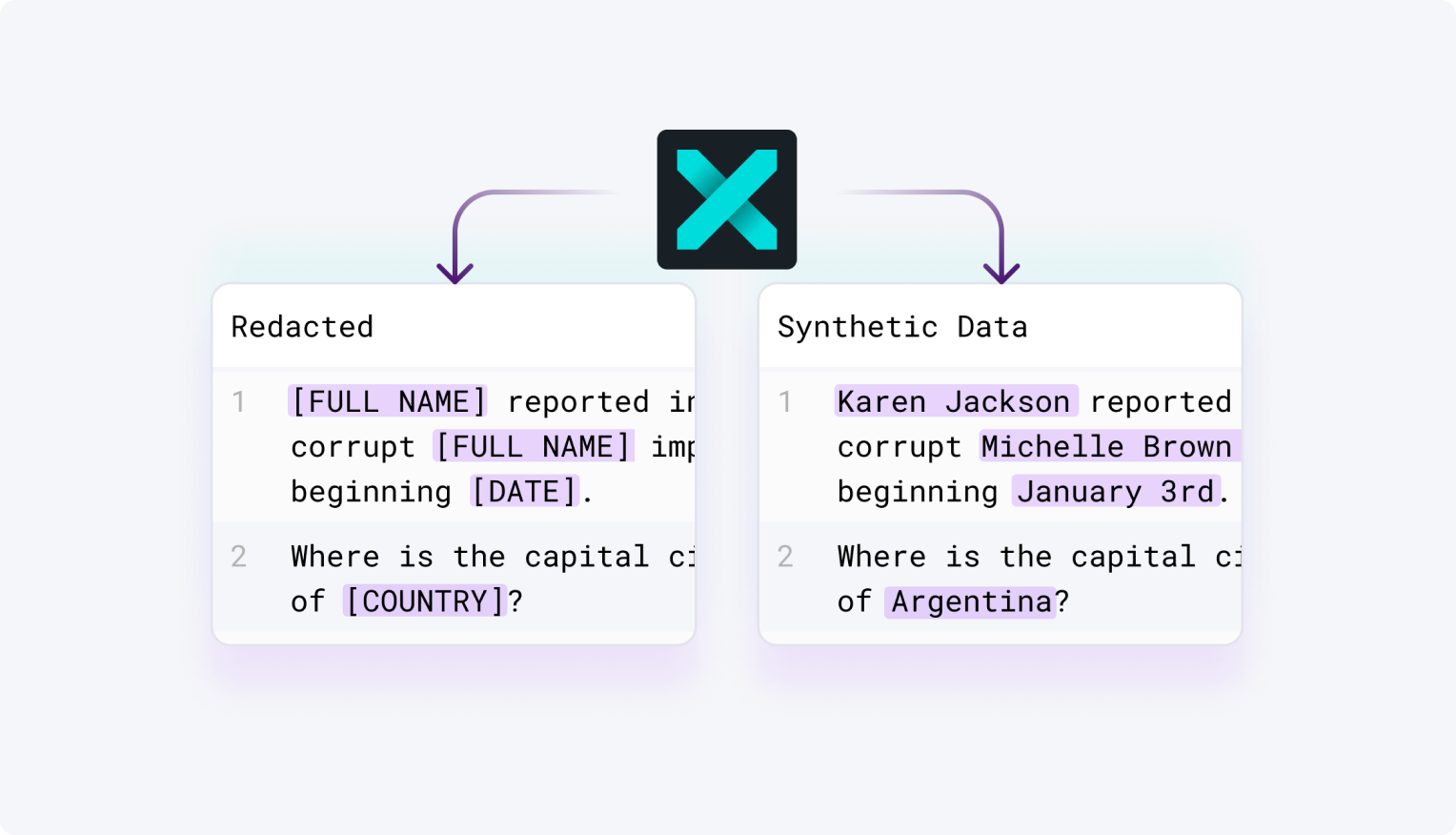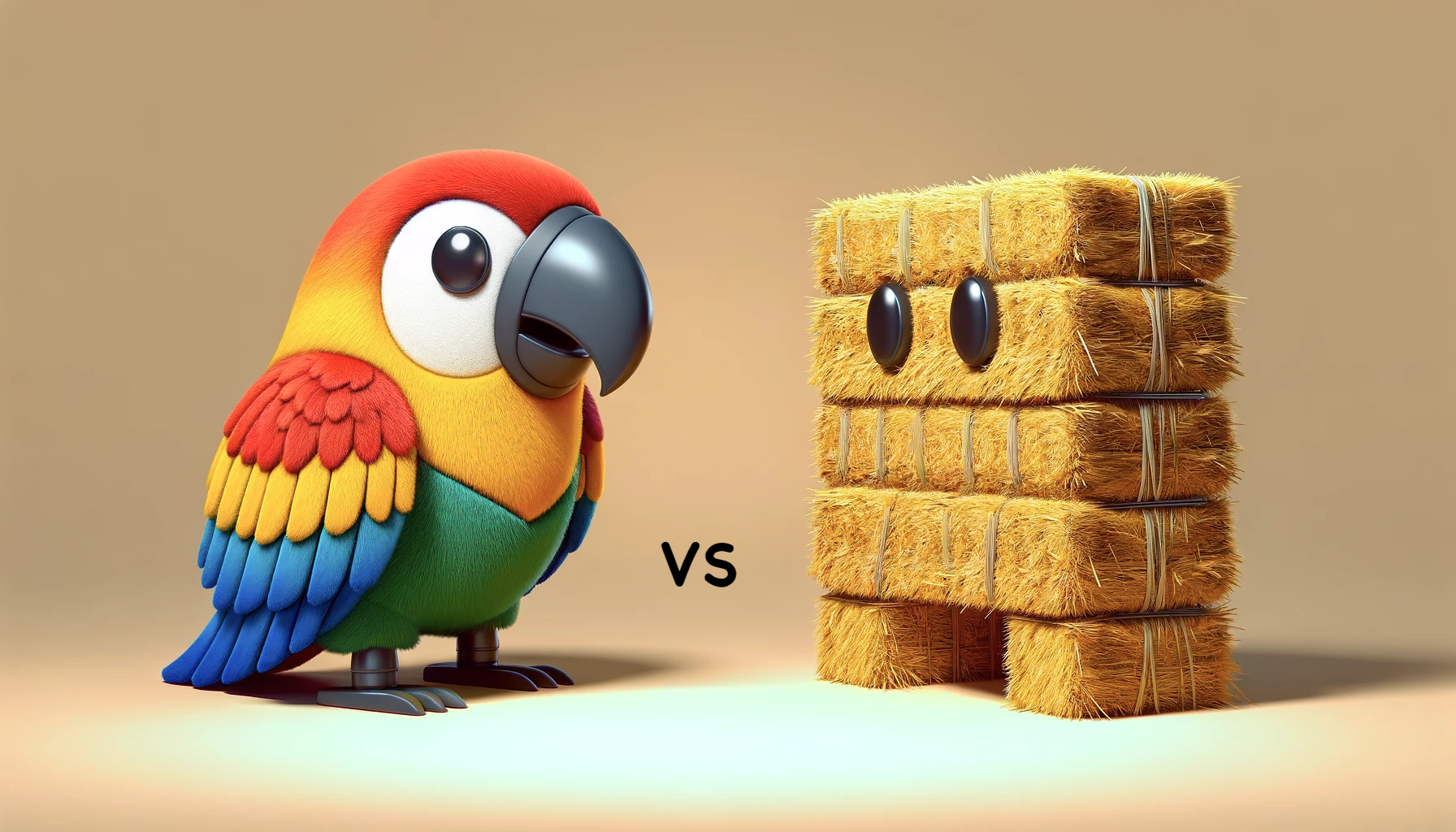Blog
Technical

# Using Neural Networks to Synthesize Complex Data Relationships, with AI SynthesizerAuthor
Joe Ferrara, PhD
November 23, 2021TL;DR: AI Synthesizer is Tonic.ai’s deep neural network generative model used to create synthetic data from a table of real data while preserving the statistical integrity of the real data. In this post, the capabilities of AI Synthesizer are shown on the King County House dataset. To evaluate the quality of the synthetic data generated by AI Synthesizer, we make statistical and machine learning model comparisons between the original data and the synthetic data. The end result? Synthetic data with a high degree of statistical similarity to real production data, great for data science use cases or wherever you need to approximate the richness of information contained in real-world datasets.

## AI Synthesizer and Evaluating Synthetic Data

If you’re new to Tonic.ai, our platform combines data anonymization with data synthesis to enable you to create fake data that mimics your production data. Among our newest features, AI Synthesizer is a machine learning generator used to create synthetic data that is as similar to the underlying real data as possible without being an exact duplicate. The architecture under the hood of the AI Synthesizer generator is that of a Variational Autoencoder (VAE).

The VAE architecture enables AI Synthesizer to create rich synthetic data from real data by approximating the multivariate joint probability distribution of the real data. AI Synthesizer preserves the distributions of the continuous variables, category frequencies of the categorical variables, and the complex relationships between all the variables. In addition to handling continuous and categorical variables, AI Synthesizer can also handle date columns and location columns in the form of longitudes and latitudes. The King County House dataset has all four of these column types (continuous, categorical, date, and location), making it a good case study to evaluate the data generated by AI Synthesizer.

There are two main concerns when evaluating the quality of synthetic data: statistical similarity and privacy. Statistical similarity refers to how statistically similar the synthetic data is to the real data. Privacy refers to how private the synthetic data is with regards to whether individual records in the real data can be identified by examining the synthetic data. These two properties are in tension as increasing one decreases the other.

In practice, the requirements of the synthetic data’s use case determines how much to value statistical similarity versus privacy. In this post, we’ll focus primarily on measuring how statistically similar the synthetic data generated by AI Synthesizer is to the original.

We use two main methods to evaluate the synthetic data generated by AI Synthesizer. The first method is to compare the distributions of the real and synthetic data. This consists of comparing histograms and correlations for the continuous variables, category frequencies for the categorical variables, and scatter plots for the longitude and latitude variables. The second method of evaluation is to train machine learning models on the synthetic and real datasets and then compare the trained models.

## The Dataset

Each row of the King County House dataset corresponds to a house that was sold in King County between May 2, 2014 and May 27, 2015. King County is home to the city of Seattle in Washington state. The dataset has 21,613 rows and 21 columns. Ignoring the id column because it is not relevant for this analysis, we broke the other 20 columns into the following categories for training the AI Synthesizer generator:

• Continuous: price, bedrooms, bathrooms, sqft_living, sqft_lot, sqft_above, sqft_basemenet, sqft_living15, sqft_lot15
• Categorical: condition, floors, grade, view, waterfront, yr_built, yr_renovated, zip code
• Location: lat, long
• Date: date (=> days_from_start)

For those of you with a particular interest in generating realistic dates (aka, all of us), here’s a quick rundown of how we handle the date column. AI Synthesizer creates a days_from_start column that, for a given date, calculates the number of days from the first date in the dataset to that date. Then the days_from_start column is treated by the VAE as a continuous variable. The synthetic days_from_start column output by the VAE is then converted back to dates to create the synthetic date column. Handling dates this way assumes the rows of the original dataset are independent and identically distributed. By independent, we mean that the information in any given row does not affect or impact information in any other row. And by identically distributed, we mean that if you think of each row as a sample from a probability distribution, then each row is coming from the same probability distribution. It’s worth noting that event data dates should not be handled this way because generating time series data is a whole ‘nother can of worms.

## Distribution Comparisons

For the distribution comparisons, the AI Synthesizer generator was trained on the 20 aforementioned columns and the whole 21,613 rows of the dataset.

### Continuous Variables

Let’s start by comparing histograms of the continuous variables. To compare the histograms, we’ll inlay the real and synthetic data histograms on top of each other. The real data is purple, the synthetic data is turquoise, and the overlap of the real and synthetic data is blue.

These inlaid histograms show that AI Synthesizer captures the distributions of the raw data — including complicated multimodal distributions.

An important aspect of AI Synthesizer is not just its ability to learn the distribution of each individual column, but also to learn the relationships between columns. One way to measure this is to compare correlations between columns, like this:

The mirrored distributions of color reflect the accuracy of how AI Synthesizer captured relationships between the columns.

### Categorical Variables

For categorical variables, we compare the category frequencies. Below are the frequency bar plots for several of the categorical variables. From the first three plots (condition, floors, and view), we see that AI Synthesizer is capable of generating similar distributions of categorical data, even in the case of highly unbalanced distributions. The final two plots (yr_built and grade) highlight the ability of the model to learn distributions of discrete data even in the presence of a large number of categories.

### Location Variables

For the latitude and longitude variables, to compare the real and synthetic data, we’ve plotted the longitude and latitude values on the x-axis and y-axis, respectively, and colored the zip codes. Below are the images. Since zip code is treated as a categorical variable, the set of zip codes in the real and synthetic data is the same, enabling the zip code colors in the two images correspond.

The synthetic data does a good job of representing the relationships between the long, lat, and zip code columns. The overall shape of the region is correct, and most zip codes are in the correct location. Also, keep in mind that AI Synthesizer was trained on all 20 columns in the dataset. If AI Synthesizer was trained only on the long, lat, and zip code columns, the above image of the synthetic data would be even more precise. As the number of columns increases, there is a decrease in the ability of the synthetic data to reconstruct the relationships between any subset of columns due to the curse of dimensionality. *shakes fist to the sky*

## Machine Learning Model Comparison

### A Multitude of Models

For our machine learning model comparisons, we performed the following process:

1. Split the real data into a training (80%) and test (20%) set. We call these two sets of data the real data training set and the (real data) test set.
2. Use AI Synthesizer to generate a synthetic data set from the real data training set. This synthetic data set is made to have the same size as the real data training set. In this step AI Synthesizer does not see the test set.
3. Given a machine learning model, train one instance of the model on the real data training set and train a separate instance of the model on the synthetic data. These two models will be called the real model and the synthetic model respectively.
4. Evaluate the real model and synthetic model on the test set, and compare the overall properties of each model.

We applied the above process to the following model types using either their default model parameters, or in the case of the MLPRegressor, slight modifications to the default parameters.

In the King County House dataset, the price column is a natural target variable, so that was used as the target variable in this exercise. All the other columns (aside from the id column) were used as input variables.

The following figure and table show the median and 95% confidence intervals of the R squared (R2) scores of the trained models after bootstrap resampling the residuals on the test set 10,000 times. The R2 score of a model is the percent of variance of the output variable (in this case price) captured by the model predictions. The closer to 1, the better the R2 score. We used R2 scores in this exercise for two reasons. First, they are a robust measurement of model performance. And second, the scale of the R2 score is independent of the data, i.e. 1 is the best score no matter what the data looks like.

In all of the model cases, there is only a small decrease in performance from the real model to the synthetic model, which is to be expected. It is also the case that across the many different model types, the decrease in performance is similar. This shows that the synthetic data is not biased towards one type of model performing better than another.

Let’s take a closer look at the CatBoost model by visualizing the price predictions. Below are the scatter plots of the price predictions of the real and synthetic CatBoost models on the test set. The price predictions are parametrized by the actual price on the x-axis, allowing us to visually see the residuals of the predictions as the price varies. In other words, in the below images there is one colored dot for each price prediction and one black dot for each actual price. The closer the colored dots are to the black dots, the better the prediction.

With regards to the synthetic versus real data comparison, as seen in the images, the heteroscedasticity (how the colored dots are spread out) of the residuals of the two models is the same. This is a good thing. It shows that the real model and synthetic model are performing similarly across different price points.

### Least Squares Coefficients

To do a least squares comparison, we trained an ordinary least squares model on the entire original dataset and the entire synthetic dataset eschewing the train test paradigm used in the previous two sections. This was done because, for ordinary least squares, we are interested in how the coefficients of the synthetic model and real model correspond, not necessarily how the models perform on a test set. Below are the 95% confidence intervals of the coefficients of the real and synthetic ordinary least squares models.

Aside from a couple outliers, the two models have similar 95% coefficient confidence intervals, which have the same sign (positive or negative). This shows that when approximated by a hyperplane, the real and synthetic models look similar, giving further evidence of the statistical similarity of the two datasets.

## The Takeaway

AI Synthesizer is a sophisticated generative model used to create protected synthetic data that is very statistically similar to real production data. It can be applied to continuous, categorical, location, and date columns when the rows of a table are independent and identically distributed. The AI Synthesizer generator is great for data science use cases of synthetic data, or in general any use case where the synthetic data needs to approximate the richness of information contained in real production data.Joe Ferrara, PhD
Data Science
Joe is a Senior Data Scientist at Tonic. He has a PhD in mathematics from UC Santa Cruz, giving him a background in complex math research. At Tonic, Joe focuses on implementing the newest developments in generating synthetic data.Real. Fake. Data.
Say goodbye to inefficient in-house workarounds and clunky legacy tools. The data you need is useful, realistic, safe—and accessible by way of API.
The LatestIntroducing Tonic Textual: Redact and synthesize sensitive free-text dataRAG Evaluation Series: Validating the RAG Performance of LangChain vs HaystackUnlocking Secure Data Utility: The Tonic.ai and Databricks Partnership and Integration